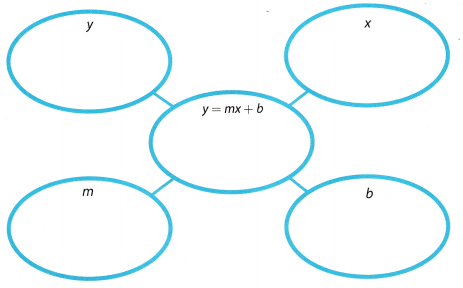# Texas Go Math Grade 8 Module 5 Answer Key Writing Linear Equations

Refer to our Texas Go Math Grade 8 Answer Key Pdf to score good marks in the exams. Test yourself by practicing the problems from Texas Go Math Grade 8 Module 5 Answer Key Writing Linear Equations.

## Texas Go Math Grade 8 Module 5 Answer Key Writing Linear Equations

Essential Question
How can you use linear equations to solve real world problems?Complete these exercises to review skills you will need for this chapter.

Write each fraction as a decimal.

Question 1.
$$\frac{1}{8}$$ ________
Given,
$$\frac{1}{8}$$
Convert from fraction to the decimal.
= 1 ÷ 8
= 0.125

$$\frac{0.3}{0.5}$$ ________
Given,
$$\frac{0.3}{0.5}$$
Convert from fraction to the decimal.
$$\frac{3}{10}$$ ÷ $$\frac{5}{10}$$ = $$\frac{3}{5}$$ = 0.6

Question 3.
$$\frac{0.13}{0.8}$$ ________
Given,
$$\frac{0.13}{0.8}$$
Convert from fraction to decimal.
$$\frac{13}{10}$$ ÷ $$\frac{8}{10}$$ = $$\frac{13}{8}$$ = 1.625

Question 4.
$$\frac{0.39}{0.52}$$ ________
Given,
$$\frac{0.39}{0.52}$$
Convert from fraction to the decimal.
$$\frac{39}{100}$$ ÷ $$\frac{52}{100}$$ = $$\frac{39}{52}$$ = 0.75

Solve each equation using the inverse operation.

Question 5.
7p = 28 __________________
7p = 28 p is multiplied by 7
$$\frac{7 p}{7}$$ = $$\frac{28}{7}$$ To solve the equation, use the inverse operation, division.
p = 4

Question 6.
h – 13 = 5 ________
h – 3 = 13 is subtracted from h
h – 13 + 13 = 5 + 13 To solve the equation, use the inverse operation, addition.
h = 18

$$\frac{y}{3}$$ = -6 ______
$$\frac{y}{3}$$ = -6 y is divided by 3
$$\frac{y}{3}$$ * 3 = -6 * 3 To solve the equation, use the inverse operation, multiplication.
y = -18

Question 8.
b + 9 = 21 _________
b + 9 = 21 9 is added to b
b + 9 – 9 = To solve the equation, use the inverse operation, subtraction.
b = 12

Question 9.
c – 8 = -8 ________________
c – 8 = 8 8 is subtracted from c
c – 8 + 8 = -8 + 8 To solve the equation, use the inverse operation, addition
c = 0

Question 10.
3n = -12 _______
3n = -12
n is multiplied by 3
$$\frac{3 n}{3}$$ = $$\frac{-12}{3}$$ To solve the equation, use the inverse operation, division.
n = -4

Question 11.
-16 = m + 7 _______
-16 = m + 7 7 is added to m

-16 – 7 = m + 7 To solve the equation, use the inverse operation, subtraction.
m = -23

$$\frac{t}{-5}$$ = -5 ____
$$\frac{t}{-5}$$ = -5
As we can see t is divided by (-5), so to solve the equation, we use the inverse operation: muLtiplication.
$$\frac{t}{-5}$$ . (-5) = (-5) . (-5)
t = 25

Visualize Vocabulary

Use the ✓ words to complete the diagram. You can put more than one word in each bubble.Understand Vocabulary

Complete the sentences using the preview words.

Question 1.
A set of data that is made up of two paired variables is ____
A set of data that is made up of two paired variables is Bivariate data
Bivariate data is defined as a set of data that is made up of two o paired variables. Each value of one of the variables is paired with a value of the other variable.
By definition, a set of data that is made up of two variables is bivariate data.
Thus, the blank part of the statement is bivariate data.

Texas Go Math Grade 8 Answer Key Pdf Module 5 Question 2.
When the rate of change varies from point to point, the relationship is a ____.